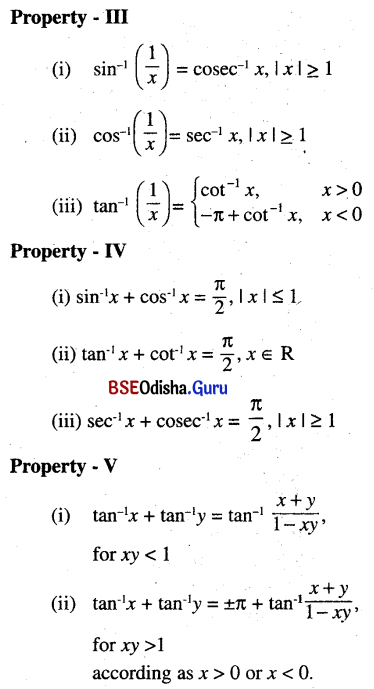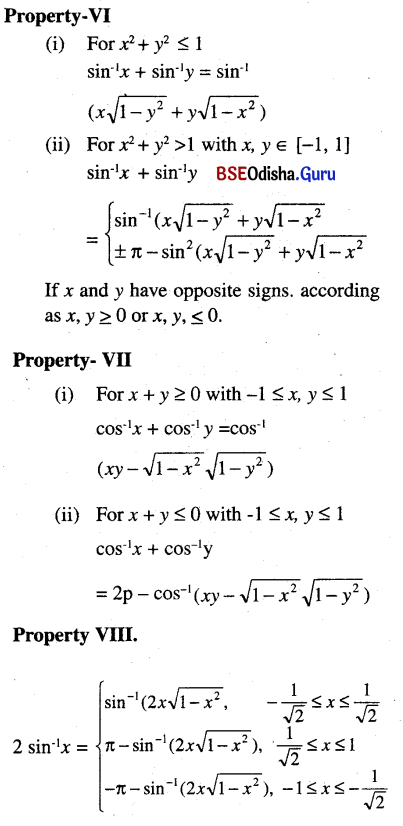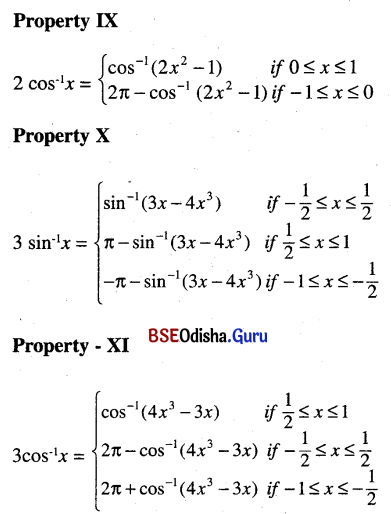# CHSE Odisha Class 12 Math Notes Chapter 2 Inverse Trigonometric Functions

Odisha State Board CHSE Odisha Class 12 Math Notes Chapter 2 Inverse Trigonometric Functions will enable students to study smartly.

## CHSE Odisha 12th Class Math Notes Chapter 2 Inverse Trigonometric Functions

Definitions:
Let us first consider the function
Sin : R → [-1, 1]
Let y = Sin x, x ∈ R. Look at the graph of sin x. For y ∈ [-1, 1], there is a unique number x in each of the intervals…, $$\left[-\frac{3 \pi}{2},-\frac{\pi}{2}\right],\left[-\frac{\pi}{2}, \frac{\pi}{2}\right],\left[\frac{\pi}{2}, \frac{3 \pi}{2}\right], \ldots$$ such that y = sin x.

Hence any one of these intervals can be chosen to make sine function bijective.

We usually choose $$\left[-\frac{\pi}{2}, \frac{\pi}{2}\right]$$ as the domain of sine function. Thus sin: $$\left[-\frac{\pi}{2}, \frac{\pi}{2}\right]$$ → [-1, 1] is bijective and hence admits of an inverse function with range $$\left[-\frac{\pi}{2}, \frac{\pi}{2}\right]$$ denoted by sin-1 or arcsin (see footnote).

Each of the above-mentioned intervals as range gives rise to different branches of sin-1 function. The function sin-1 with the range $$\left[-\frac{\pi}{2}, \frac{\pi}{2}\right]$$ is called the principal branch which is defined below.

sin-1 : [-1, 1] → $$\left[-\frac{\pi}{2}, \frac{\pi}{2}\right]\left[-\frac{\pi}{2}, \frac{\pi}{2}\right]$$ defined by y = sin-1 x ⇔ x = sin y.

The values of y (=sin-1 x) in $$\left[-\frac{\pi}{2}, \frac{\pi}{2}\right]$$ are called principal values of sin-1.

Similar considerations for other trigonometric functions give rise to respective inverse functions. We define below the principal branches of cos-1, tan-1 and cot-1.

cos-1 : [-1, 1] → [0, π] defined by
y = cos-1 x ⇔ x = cos y
tan-1 : R → $$\left[-\frac{\pi}{2}, \frac{\pi}{2}\right]$$ defined by
y = tan-1 x ⇔ x = tan y
cot-1 : R → (0, π) defined by
y = cot-1 x ⇔ x = cot yImportant Properties

Property – I
We know that when
f : X → Y is invertible then fof-1 = Iy and f-1of = Ix.
Applying this we have.
(i) sin (sin-1 x) = x, x ∈ [-1, 1]
cos (cos-1 x) = x, x ∈ [-1, 1]
tan (tan-1 x) = x, x ∈ R
cot (cot-1 x) = x, x ∈ R
sec (sec-1 x) = x, x ∈ R -(-1, 1)
cosec (cosec-1 x) = x, x ∈ R -(-1, 1)

Property – II
(i) sin-1(-x) = -sin-1 x, x ∈ [-1, 1]
(ii) cosec-1(-x) = -cosec-1 x , |x| ≥ 1
(iii) tan-1(-x) = -tan-1 x, x ∈ R